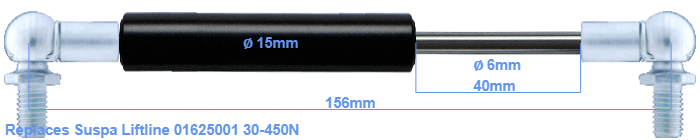# Replacement for Suspa Liftline 01625001 30-450N

£27.05 (excl. VAT)

Replacement gas spring for the Suspa Liftline 01625001 30-450 Newton. This fits a (possibly already present) ball with a diameter of 10mm. A corresponding ball is included. Brand: Hahn.
 Force Choose an option30 Newton40 Newton50 Newton60 Newton80 Newton100 Newton120 Newton140 Newton150 Newton160 Newton180 Newton200 Newton220 Newton240 Newton250 Newton260 Newton280 Newton300 Newton320 Newton340 Newton350 Newton360 Newton380 Newton400 Newton420 Newton440 Newton450 NewtonClear
This gas spring is also known as 16-1 016 25001, 16-1-72,5-45-A246-B246.